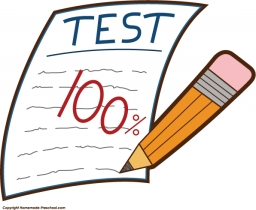# Probability 40131

There are 28 students in the IK3 class. Three pupils will be examined. Nineteen pupils are ready for the exam. What is the probability that all three will be unprepared?

p =  0.0256

### Step-by-step explanation:Did you find an error or inaccuracy? Feel free to write us. Thank you!

Math student
This is incorrect as it assumes a constant probability as the kids are chosen. The answer would be 9/28 * 8/27 * 7/26 OR 9C3 / 28C3.

Matik
Hello, we corrected this

Tips for related online calculators
Need help calculating sum, simplifying, or multiplying fractions? Try our fraction calculator.
Would you like to compute the count of combinations?